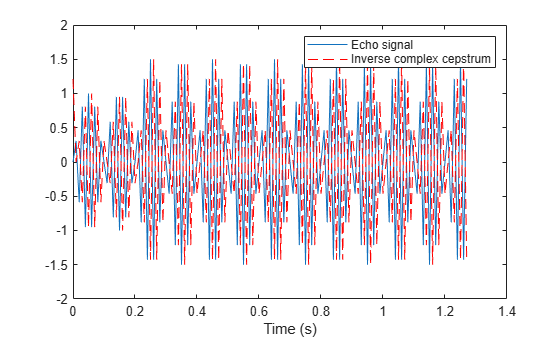Documentation

# icceps

Inverse complex cepstrum

## Syntax

``x = icceps(xhat,nd)``

## Description

example

````x = icceps(xhat,nd)` returns the inverse complex cepstrum of the real data sequence `xhat`, removing `nd` samples of delay.```

## Examples

collapse all

Generate a sine of frequency 45 Hz, sampled at 100 Hz. Add an echo with half the amplitude and 0.2 s later. Compute the complex cepstrum of the signal.

```Fs = 100; t = 0:1/Fs:1.27; s1 = sin(2*pi*45*t); s2 = s1 + 0.5*[zeros(1,20) s1(1:108)]; c = cceps(s2);```

Compute the inverse complex cepstrum. Plot the echo data and its inverse complex cepstrum.

```x = icceps(c); plot(t,s2,t,x,'r--') xlabel('Time (s)') legend('Echo signal','Inverse complex cepstrum')```## Input Arguments

collapse all

Data sequence, specified as a real vector. If `xhat` was obtained with `cceps`, then the amount of delay that was added to `x` was the element of `round(unwrap(angle(fft(x)))/pi)` corresponding to π radians.

Number of samples of delay, specified as a real positive scalar.

## Output Arguments

collapse all

Inverse complex cepstrum, returned as a vector.

 Oppenheim, A.V., and R.W. Schafer, Discrete-Time Signal Processing, Prentice-Hall, 1989.